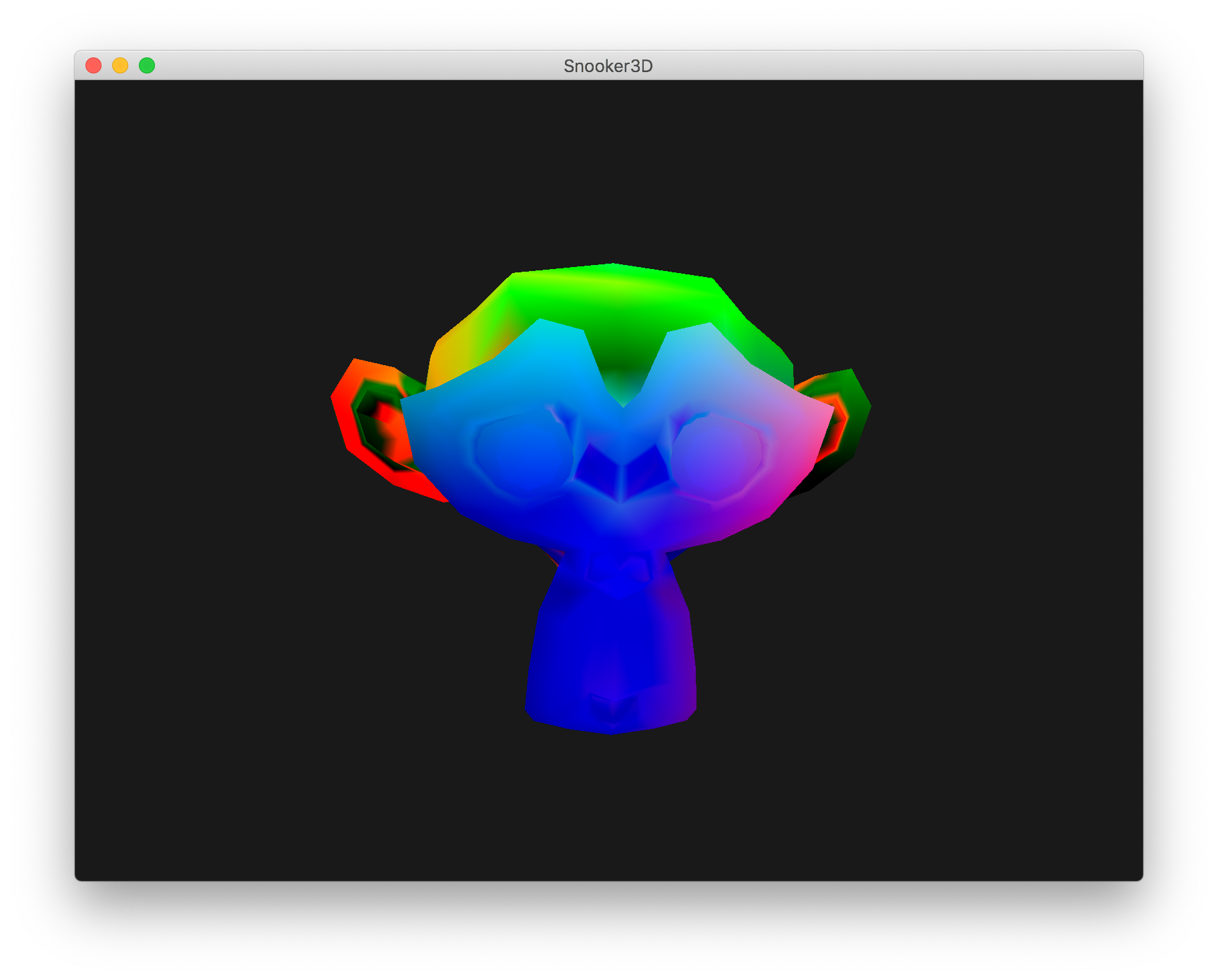A semi-curated blog of computer graphics and rendering.

This is a blog post from the past. Originally written at 2019-12-10.

Model loading is a very important aspect in Computer Graphics. Sometimes you really need something with a good-looking pattern, you know. Then we got assimp, which is great for model loading and also bulges in size. So what’s the best substitution? tinyobjloader, I wager.

Tiny but powerful single file wavefront obj loader written in C++03. No dependency except for C++ STL. It can parse over 10M polygons with moderate memory and time.

The author of this project is a GI maniac. I like him! Let’s get started!

## Getting Started

This is a single header file library just like the rest. The source file is here. You can download its .cc file too if you want a static library. But there really isn’t much difference here!

Well, once that file is here, we can begin loading the model. It’s pretty important to define a helper class first:

class Vertex {
public:
glm::vec3 position;
glm::vec3 normal;
glm::vec2 texCoord;
};


Oh yeah, we also use glm and C++. Take it or leave it. This one’s simple, right?

std::vector<tinyobj::shape_t> shapes;
std::vector<tinyobj::material_t> materials;
std::string warnings;
std::string errors;
tinyobj::LoadObj(&attributes, &shapes, &materials, &warnings, &errors, modelPath.c_str(), modelMtlBaseDir.c_str());


And all attributes will go straight into attribuets; shapes go into shapes, etc. The attribute variable hosts all vertex data: vertex, normals, etc.

Now, as this indice is a little bit different in OpenGL - its position, texture coordinate & normals’ indices are stored separated - we have the liberty to either reform a new array of union coordinate and a union index, or just be lazy and decompress the indices and form a rather large & space-wasting buffer, which saves time. Let’s do the latter one!

## Decompress!

The procedure of decompression is not hard. Actually, it’s fine. Let’s sort through it.

Tips: I am using a single buffer here (because of the laziness). As every shape repersents a mesh, you could use multiple buffers. Just do whatever fits your taste!

First, we define variables:

std::vector<Vertex> vertices;


Just one! Isn’t this handy?

Then, we iterate through shapes:

for (int i = 0; i < shapes.size(); i ++) {
tinyobj::shape_t &shape = shapes[i];
tinyobj::mesh_t &mesh = shape.mesh;
// we could visit the mesh index by using mesh.indices
...
}


In this way, we could visit all meshes separately. As I said earlier, you can split them into multiple vertex arrays. No rush. I was using only one because I am lazy.

In this loop, we should iterate over all mesh index and create corresponding vertex.

// Replace the ... above
for (int j = 0; j < mesh.indices.size(); j++) {
tinyobj::index_t i = mesh.indices[j];
glm::vec3 position = {
attributes.vertices[i.vertex_index * 3],
attributes.vertices[i.vertex_index * 3 + 1],
attributes.vertices[i.vertex_index * 3 + 2]
};
glm::vec3 normal = {
attributes.normals[i.normal_index * 3],
attributes.normals[i.normal_index * 3 + 1],
attributes.normals[i.normal_index * 3 + 2]
};
glm::vec2 texCoord = {
attributes.texcoords[i.texcoord_index * 2],
attributes.texcoords[i.texcoord_index * 2 + 1],
};
// Not gonna care about texCoord right now.
Vertex vert = { position, normal, texCoord };
vertices.push_back(vert);
}
...


Well, that wasn’t hard, no? In fact, you can check whether the index’s normal or texture coordinate exists by using if (i.vertex_index < 0). You get the gist.

Anyway, now after this loop, the vertices array should be bulging with vertices already. And… That’s it! The model has been loaded. You could initialize the VAO like this:

GLuint VAO;
glGenVertexArrays(1, &VAO);
glBindVertexArray(VAO);
GLuint VBO;
glGenBuffers(1, &VBO);
glBindBuffer(GL_ARRAY_BUFFER, VBO);
glBufferData(GL_ARRAY_BUFFER, sizeof(Vertex) * vertices.size(), &vertices, GL_STATIC_DRAW);
glEnableVertexAttribArray(0);
glVertexAttribPointer(0, 3, GL_FLOAT, GL_FALSE, sizeof(float) * 8, nullptr);
glEnableVertexAttribArray(1);
glVertexAttribPointer(1, 3, GL_FLOAT, GL_FALSE, sizeof(float) * 8, (void *) (sizeof(float) * 3));
glEnableVertexAttribArray(2);
glVertexAttribPointer(2, 2, GL_FLOAT, GL_FALSE, sizeof(float) * 8, (void *) (sizeof(float) * 6));


And render it like this:

glBindVertexArray(VAO);
glDrawArrays(GL_TRIANGLES, 0, vertices.size());


## What, that’s it?

Yeah! As I said, I was being lazy. Take it or leave it. This is a very powerful library. If you want indexed rendering, you could totally do that. At the mean time, please check out the examples the library comes with. Particularly this one is very helpful. I am sorry I don’t have a lot of time & efficiency and gentleness isn’t my top priority. I will dig into it in the future should I have time. At the meantime, isn’t this great?!

Oh yeah, seems like I forgot to cover that. Well, as texture rendering isn’t what I need right now, I really didn’t look into it. However feel free to dig into the example source! It comes with texture rendering.

OK, here it is:

tinyobj::attrib_t attributes;
std::vector<tinyobj::shape_t> shapes;
std::vector<tinyobj::material_t> materials;
std::string warnings;
std::string errors;

tinyobj::LoadObj(&attributes, &shapes, &materials, &warnings, &errors, modelPath.c_str(), modelMtlBaseDir.c_str());

std::vector<Vertex> vertices;
for (int i = 0; i < shapes.size(); i ++) {
tinyobj::shape_t &shape = shapes[i];
tinyobj::mesh_t &mesh = shape.mesh;
for (int j = 0; j < mesh.indices.size(); j++) {
tinyobj::index_t i = mesh.indices[j];
glm::vec3 position = {
attributes.vertices[i.vertex_index * 3],
attributes.vertices[i.vertex_index * 3 + 1],
attributes.vertices[i.vertex_index * 3 + 2]
};
glm::vec3 normal = {
attributes.vertices[i.normal_index * 3],
attributes.vertices[i.normal_index * 3 + 1],
attributes.vertices[i.normal_index * 3 + 2]
};
glm::vec2 texCoord = {
attributes.vertices[i.texcoord_index * 2],
attributes.vertices[i.texcoord_index * 2 + 1],
};
Vertex vert = { position, normal, texCoord };
vertices.push_back(vert);
}
}

GLuint VAO;
glGenVertexArrays(1, &VAO);
glBindVertexArray(VAO);
GLuint VBO;
glGenBuffers(1, &VBO);
glBindBuffer(GL_ARRAY_BUFFER, VBO);
glBufferData(GL_ARRAY_BUFFER, sizeof(Vertex) * vertices.size(), &vertices, GL_STATIC_DRAW);
glEnableVertexAttribArray(0);
glVertexAttribPointer(0, 3, GL_FLOAT, GL_FALSE, sizeof(float) * 8, nullptr);
glEnableVertexAttribArray(1);
glVertexAttribPointer(1, 3, GL_FLOAT, GL_FALSE, sizeof(float) * 8, (void *) (sizeof(float) * 3));
glEnableVertexAttribArray(2);
glVertexAttribPointer(2, 2, GL_FLOAT, GL_FALSE, sizeof(float) * 8, (void *) (sizeof(float) * 6));


Well, that’s pretty much it. Good luck, and farewell!

Finally, an image of our beloved monkey, suzanne, rendered in normal as its skin color: